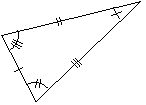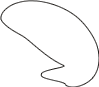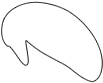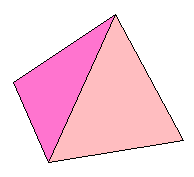index: click on a letter A B C D E F G H I J K L M N O P Q R S T U V W X Y Z A to Z index index: subject areas numbers & symbols sets, logic, proofs geometry algebra trigonometry advanced algebra & pre-calculus calculus advanced topics probability & statistics real world applications multimedia entrieswww.mathwords.com about mathwords website feedback

Congruent

Exactly equal in size and shape. Congruent sides or segments have the exact same length. Congruent angles have the exact same measure. For any set of congruent geometric figures, corresponding sides, angles, faces, etc. are congruent.

Note: Congruent segments, sides, and angles are often marked.

 Congruent Segments Congruent AnglesAll four sides of this rhombus are marked to show they are congruent to each other.The marked angles of this isosceles triangle are congruent to each other.

 Congruent TrianglesThese triangles are congruent. Corresponding sides and angles are congruent. Congruent Plane FiguresThese figures are congruent because their shapes and sizes are identical, even though there are no sides or angles to measure and compare. Congruent PyramidsThese are congruent figures because their sizes and shapes are identical. Note that corresponding faces of these pyramids are congruent.